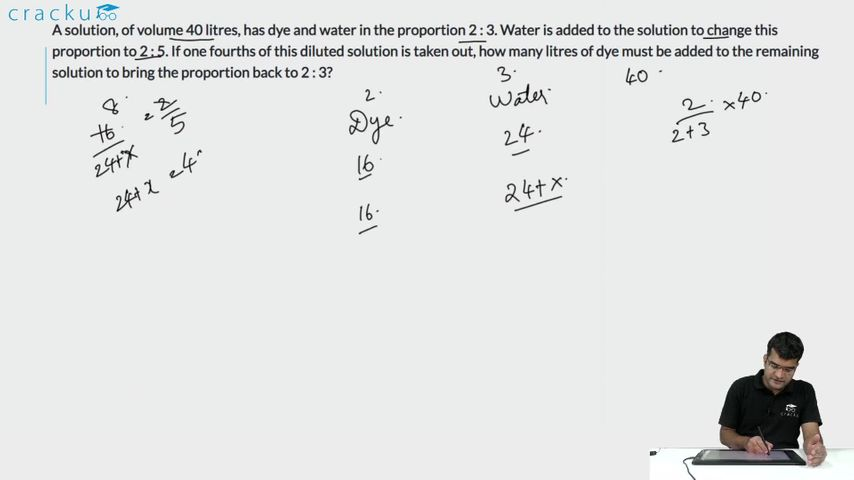Question 13

# A solution, of volume 40 litres, has dye and water in the proportion 2 : 3. Water is added to the solution to change this proportion to 2 : 5. If one fourths of this diluted solution is taken out, how many litres of dye must be added to the remaining solution to bring the proportion back to 2 : 3?

Solution

Initially the amount of Dye and Water are 16,24 respectively.

To make the ratio of Dye to Water to 2:5 the amount of water should be 40l for 16l of Dye=> 16l of water is added.

Now, the Dye and Water arr 16,40 respectively.

After removing 1/4th of solution the amount of Dye and Water will be 12,30l respectively.

To have Dye and Water in the ratio of 2:3, for 30l of water we need 20l of Dye => 8l of Dye should be added.

Hence , 8 is correct answer.

### View Video Solution